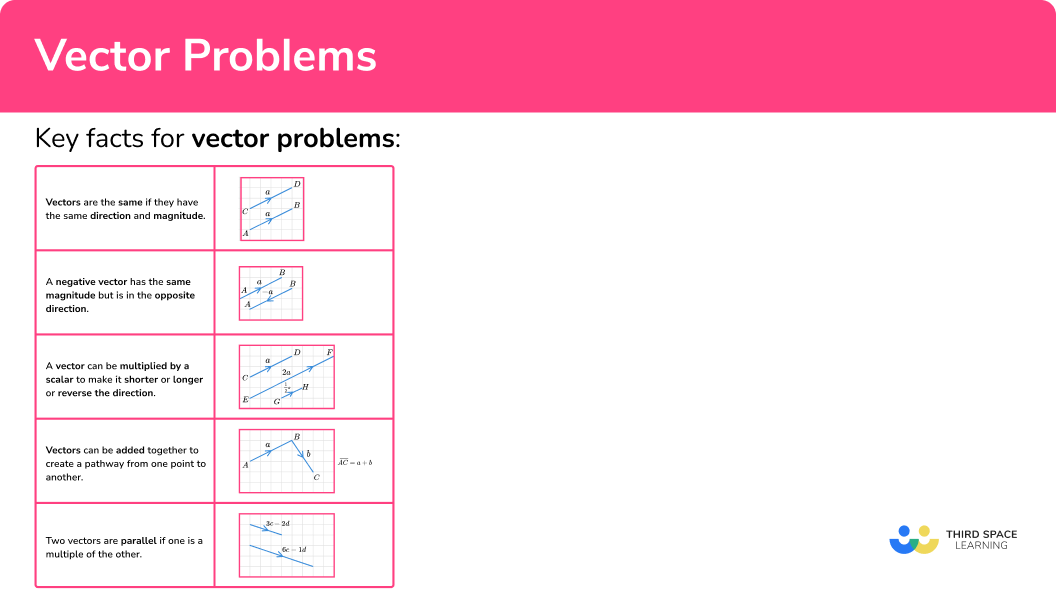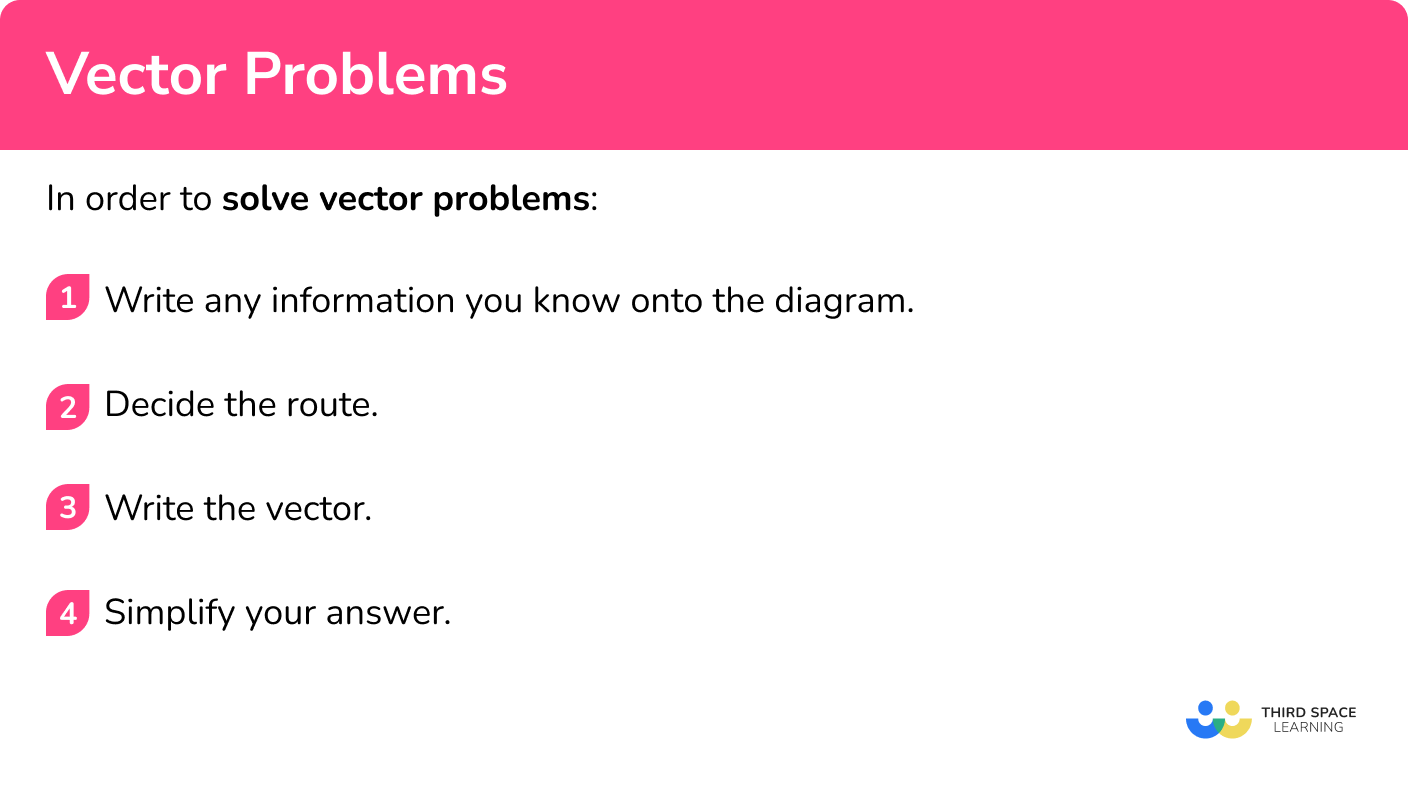# Vector Problems

Here we will learn about more difficult vector problems, including vector routes involving midpoints, fractions and ratios of lengths. We will also look at parallel vectors.

There are also vector worksheets based on Edexcel, AQA and OCR exam questions, along with further guidance on where to go next if you’re still stuck.

## What are vector problems?

Vector problems use vectors to solve a variety of different types of problems. Vectors have both a magnitude and direction and can be used to show a movement. A quantity which has just magnitude (size) is called a scalar.

For example,

We can write vectors in several ways,

• Using an arrow
• Using boldface
• Underlined

\overrightarrow{AB} =\textbf{a}=\underline{a}

### What are vector problems?## Key facts for vector problems

In order to solve problems involving vectors it is helpful to use several key facts.

## How to solve vector problems

In order to solve vector problems:

1. Write any information you know onto the diagram.
2. Decide the route.
3. Write the vector.

### Explain how to solve vector problems## Related lessons on vectors

Vector problems is part of our series of lessons to support revision on vectors. You may find it helpful to start with the main vectors lesson for a summary of what to expect, or use the step by step guides below for further detail on individual topics. Other lessons in this series include:

## Vector problem examples

### Example 1: parallel lines

The shape below is made from 8 equilateral triangles.

\overrightarrow{PQ} = \textbf{a},~ \overrightarrow{OQ} = \textbf{b}

Find the vector \overrightarrow{QT} .

1. Write any information you know onto the diagram.

Parallel vectors of the same magnitude are the same. These triangles are all identical therefore we can label the corresponding vectors a and b .

2Decide the route.

We need to find a route where we know the vectors.

3Write the vector.

\overrightarrow{QT}=-\textbf{b}-\textbf{b}+\textbf{a}-\textbf{b}

\overrightarrow{QT}=\textbf{a}-3\textbf{b}

### Example 2: extended line

\overrightarrow{AC}=\textbf{a}, ~\overrightarrow{BC}=\textbf{b}

The line AB is extended to the point D so that the length AD is three times the length AB .

There is currently no further information we can add to the diagram.

We know AD is three times the length of AB . We need to find a route from A to B and then multiply it by three.

### Example 3: midpoint

\overrightarrow{BC} = \textbf{p}, ~\overrightarrow{BA}=\textbf{q}

D is the midpoint of AC . Find the vector \overrightarrow{BD} .

When we are given information about midpoints, fractions of lines or ratios, it can be helpful to add this information onto the diagram. Here we are going to use the fraction  \frac{1}{2} to show that D is half way along the line.

We are trying to find a route from B to D . We know that \overrightarrow{BC}=\textbf{p} and we then need to get from C to D . We also know that CD=\frac{1}{2}CA .

We can find the vector \overrightarrow{CA} and then half it.

\begin{aligned} &\overrightarrow{CA}=-\textbf{p}+\textbf{q}\\\\ &\overrightarrow{CD}=\frac{1}{2}\overrightarrow{CA}=\frac{1}{2}(-\textbf{p}+\textbf{q}) \end{aligned}

Now we have a route from B to D .

\overrightarrow{BD}=\textbf{p}+\frac{1}{2}(-\textbf{p}+\textbf{q})

\begin{aligned} &\overrightarrow{BD}=\textbf{p}+\frac{1}{2}(-\textbf{p}+\textbf{q})\\\\ &\overrightarrow{BD}=\textbf{p}-\frac{1}{2}\textbf{p}+\frac{1}{2}\textbf{q}\\\\ &\overrightarrow{BD}=\frac{1}{2}\textbf{p}+\frac{1}{2}\textbf{q} \end{aligned}

### Example 4: fraction of a line

\begin{aligned} &\overrightarrow{AB} = 2\textbf{a}, ~ \overrightarrow{AC} =6\textbf{b}\\\\ &\text{BM }=\frac{1}{4}\text{ BA}, \text{ AN} = \frac{1}{3} \text{ AC} \end{aligned}

Find the vector \overrightarrow{MN} .

When we are given information about midpoints, fractions of lines or ratios, it can be helpful to add this information onto the diagram. Here we know

\text{BM }=\frac{1}{4}\text{ BA}, \text{ AN} = \frac{1}{3} \text{ AC} .

We need to find a route from M to N . We can see that

\text{MA}=\frac{3}{4}\text{BA and AN}=\frac{1}{3}\text{AC} .

\overrightarrow{MA}=\frac{3}{4}\overrightarrow{BA}=\frac{3}{4}(-2\textbf{a})=-\frac{3}{2}\textbf{a}\\\overrightarrow{AN}=\frac{1}{3}\overrightarrow{AC}=\frac{1}{3}(6\textbf{b})=2\textbf{b}

We now have a route from M to N .

\overrightarrow{MN}=-\frac{3}{2}\textbf{a}+2\textbf{b}

The answer here cannot be simplified.

### Example 5: ratio

\overrightarrow{FG} =\textbf{a}, ~ \overrightarrow{EH} =2\textbf{a}, ~ \overrightarrow{EF} =\textbf{b}

ED =2EH and the point J is such that GJ:JH = 2:1 .

Find the vector \overrightarrow{JD} .

When we are given information about midpoints, fractions of lines or ratios, it can be helpful to add this information onto the diagram. Here we know GJ:JH=2:1 . This means that  \text{GJ}=\frac{2}{3}\text{GH and JH}=\frac{1}{3}\text{GH} .

We also know that ED=2EH therefore HD is the same length as EH and in the same direction.

We know  \text{JH}=\frac{1}{3}\text{GH} .

We can find the vector  \overrightarrow{GH} .

\begin{aligned} &\overrightarrow{GH}=-\textbf{a}-\textbf{b}+2\textbf{a}=\textbf{a}-\textbf{b}\\\\ &\overrightarrow{JH}=\frac{1}{3}\overrightarrow{GH}=\frac{1}{3}(\textbf{a}-\textbf{b}) \end{aligned}

We now have a route from J to D .

\overrightarrow{JD}=\frac{1}{3}(\textbf{a}-\textbf{b})+2\textbf{a}

\begin{aligned} &\overrightarrow{JD}=\frac{1}{3}(\textbf{a}-\textbf{b})+2\textbf{a}\\\\ &\overrightarrow{JD}=\frac{1}{3}\textbf{a}-\frac{1}{3}\textbf{b}+2\textbf{a}\\\\ &\overrightarrow{JD}=\frac{7}{3}\textbf{a}-\frac{1}{3}\textbf{b} \end{aligned}

### Example 6: mix of information

\overrightarrow{AB} =6\textbf{a}, ~ \overrightarrow{BC} =10\textbf{b}

E is the point on AB such that \text{EB }=\frac{1}{3}\text{ AB} .

D is the point on BC such that CD:DB=1:4 .

F is the midpoint of ED .

Find the vector \overrightarrow{EF} .

We want to find the the vector \overrightarrow{EF} .

We know that \text{EF}=\frac{1}{2}\text{ED} so we can start by finding the vector \overrightarrow{ED} .

\begin{aligned} &\overrightarrow{EB}=\frac{1}{3}\overrightarrow{AB}=\frac{1}{3}(6\textbf{a})=2\textbf{a}\\\\ &\overrightarrow{BD}=\frac{4}{5}\overrightarrow{BC}=\frac{4}{5}(10\textbf{b})=8\textbf{b} \end{aligned}

\overrightarrow{ED}=2\textbf{a}+8\textbf{b}

\overrightarrow{EF}=\frac{1}{2}\overrightarrow{ED}=\frac{1}{2} (2\textbf{a}+8\textbf{b})=\textbf{a}+4\textbf{b}

## How to show two vectors are parallel

Two vectors are parallel if one is a multiple of the other. This is because if one vector is a multiple of another, it is a bigger or smaller version of the other.

In order to show two vectors are parallel:

1. Work out each vector.
2. Show that one is a multiple of the other by factorising.

## Showing two vectors are parallel examples

### Example 7: two lines are parallel

Show that AB is parallel to CD .

\overrightarrow{CD}=\textbf{a}+2\textbf{b}

We need to find the vector \overrightarrow{AB} .

\begin{aligned} &\overrightarrow{AB}=4\textbf{a}+\textbf{a}+2\textbf{b}-3\textbf{a}+2\textbf{b}\\\\ &\overrightarrow{AB}=2\textbf{a}+4\textbf{b} \end{aligned}

\overrightarrow{AB}=2\textbf{a}+4\textbf{b}=2(\textbf{a}+2\textbf{b})=2\overrightarrow{CD}

Since the vector  \overrightarrow{AB} is a multiple of the vector  \overrightarrow{CD} these vectors are parallel.

### Example 8: two line segments form one straight line

ABCD is a parallelogram.

The line AB is extended to the point E such that \text{BE}=\frac{2}{3}\text{AB} .

The point F is on the line BC such that BF:FC=2:3 .

\overrightarrow{AB}=6\textbf{a}, \overrightarrow{CB}=5\textbf{b}

Show that DFE is a straight line.

We need to find the vectors \overrightarrow{DF} \text{ and } \overrightarrow{FE} .

\begin{aligned} &\overrightarrow{CF}=\frac{3}{5}\overrightarrow{CB}=3\textbf{b}\\\\ &\overrightarrow{FB}=\frac{2}{5}\overrightarrow{CB}=2\textbf{b} \end{aligned}

\begin{aligned} &\overrightarrow{DF}=6\textbf{a}+3\textbf{b}\\\\ &\overrightarrow{FE}=4\textbf{a}+2\textbf{b} \end{aligned}

$\overrightarrow{\mathrm{DF}}=1.5(4 \mathbf{a}+2 \mathbf{b})=1.5 \overrightarrow{\mathrm{FE}}$

Since \overrightarrow{DF} is a multiple of \overrightarrow{FE} the vectors are parallel. They also share the point F meaning they form a straight line.

### Common misconceptions

• Using the wrong sign

Remember to make the vector negative when going backwards along it.

• Mistakes with ratios

If two parts of a line are in the ratio 1:4, this means one part is ⅕ of a line and the other part ⅘ .

### Practice vector problem questions

1. ABCD is a parallelogram. The line AD is extended to the point E so that AE=3AD .

Find the vector  \overrightarrow{CE} .-\textbf{p}+3\textbf{q}-\textbf{p}+\textbf{q}-\textbf{p}+2\textbf{q}\textbf{p}+2\textbf{q}\overrightarrow{CE}=-\textbf{p}+2\textbf{q}

2. \overrightarrow{PR}=4\textbf{a}, \overrightarrow{PQ}=3\textbf{b}

M is the midpoint of QR .

Find the vector \overrightarrow{RM} .-2\textbf{a}+1.5\textbf{b}-4\textbf{a}+3\textbf{b}-2\textbf{a}+3\textbf{b}2\textbf{a}-1.5\textbf{b}\text{RM}=\frac{1}{2}\text{RQ}\begin{aligned} &\overrightarrow{RQ}=-4\textbf{a}+3\textbf{b}\\\\ &\overrightarrow{RM}=\frac{1}{2}\overrightarrow{RQ}=\frac{1}{2}(-4\textbf{a}+3\textbf{b})=-2\textbf{a}+1.5\textbf{b} \end{aligned}

3. \overrightarrow{AB}=3\textbf{b}, \overrightarrow{BC}=2\textbf{a}

AD=2BC and the point E is on the line CD such that \text{ED}=\frac{1}{3}\text{CD} .

Find the vector \overrightarrow{AE} .\frac{14}{3}\textbf{a}-\textbf{b}\frac{10}{3}\textbf{a}+\textbf{b}\textbf{b}+\frac{2}{3}\textbf{a}\textbf{b}-\frac{2}{3}\textbf{a}We know \text{DC}=\frac{1}{3}\text{DC} so we need to find the vector \overrightarrow{DC} .\begin{aligned} &\overrightarrow{DC}=-4\textbf{a}+3\textbf{b}+2\textbf{a}=3\textbf{b}-2\textbf{a}\\\\ &\overrightarrow{DE}=\frac{1}{3}\overrightarrow{DC}=\frac{1}{3}(3\textbf{b}-2\textbf{a}) \end{aligned}\begin{aligned} &\overrightarrow{AE}=4\textbf{a}+\frac{1}{3}(3\textbf{b}-2\textbf{a})\\\\ &\overrightarrow{AE}=4\textbf{a}+\textbf{b}-\frac{2}{3}\textbf{a}\\\\ &\overrightarrow{AE}=\frac{10}{3}\textbf{a}+\textbf{b} \end{aligned}

4. \overrightarrow{AC}=9\textbf{a}, \overrightarrow{AB}=8\textbf{b}

The point N is such that \text{AN}=\frac{1}{3}\text{AC} , and the point P is such that AP:PB=1:3 . M is the midpoint of BN . Find the vector \overrightarrow{PM} .\frac{3}{2}\textbf{a}+2\textbf{b}\frac{3}{2}\textbf{a}+\frac{4}{3}\textbf{b}-\frac{3}{2}\textbf{a}-2\textbf{b}\frac{3}{2}\textbf{a}-2\textbf{b}We need to find a route from P to M . We can see \overrightarrow{PB}=6\textbf{b} .

We know that BM=\frac{1}{2}BN so we need to find the vector \overrightarrow{BN} .\begin{aligned} &\overrightarrow{BN}=-8\textbf{b}+3\textbf{a}\\\\ &\overrightarrow{BM}=\frac{1}{2}\overrightarrow{BN}=\frac{1}{2}(-8\textbf{b}+3\textbf{a})\\\\ &\overrightarrow{PM}=6\textbf{b}+\frac{1}{2}(-8\textbf{b}+3\textbf{a})\\\\ &\overrightarrow{PM}=6\textbf{b}-4\textbf{b}+\frac{3}{2}\textbf{a}\\\\ &\overrightarrow{PM}=\frac{3}{2}\textbf{a}+2\textbf{b} \end{aligned}

5. \overrightarrow{AB}=2\textbf{a}+6\textbf{b}, \overrightarrow{AC}=3\textbf{a}+2\textbf{b}

D is the midpoint of BC and E is the point on AD such that AE:ED=1:3 .

Find the vector \overrightarrow{AE} .\frac{5}{2}\textbf{a}+4\textbf{b}\textbf{a}-4\textbf{b}\frac{5}{8}\textbf{a}+\textbf{b}-\frac{5}{4}\textbf{a}-\frac{23}{4}\textbf{b}We need to begin by finding the vector \overrightarrow{AD} .\begin{aligned} &\overrightarrow{AB}=2\textbf{a}+6\textbf{b}\\\\ &\overrightarrow{BC}=-2\textbf{a}-6\textbf{b}+3\textbf{a}+2\textbf{b}=\textbf{a}-4\textbf{b}\\\\ &\overrightarrow{BD}=\frac{1}{2}\overrightarrow{BC}=\frac{1}{2}\textbf{a}-2\textbf{b}\\\\ &\overrightarrow{AD}=2\textbf{a}+6\textbf{b}+\frac{1}{2}\textbf{a}-2\textbf{b}=\frac{5}{2}\textbf{a}+4\textbf{b}\\\\ &\overrightarrow{AE}=\frac{1}{4}(\frac{5}{2}\textbf{a}+4\textbf{b})=\frac{5}{8}\textbf{a}+\textbf{b} \end{aligned}

6. \overrightarrow{AB}=4\textbf{a}, \overrightarrow{AE}=8\textbf{b}
D is the midpoint of AE .

Which vector is parallel to \textbf{b}-\textbf{a} ?\overrightarrow{AB}\overrightarrow{AC}\overrightarrow{CE}\overrightarrow{BE}\begin{aligned} &\overrightarrow{AB}=4\textbf{a}\\\\ &\overrightarrow{AC}=4\textbf{a}+4\textbf{b}\\\\ &\overrightarrow{CE}=4\textbf{b}-4\textbf{a}\\\\ &\overrightarrow{BE}=8\textbf{b}-4\textbf{a} \end{aligned}

4\textbf{b}-4\textbf{a}=4(\textbf{b}-\textbf{a}) so \overrightarrow{CE} is parallel to \textbf{b}-\textbf{a} .

### Vector problems GCSE questions

1. \overrightarrow{AB}=6\textbf{b},\overrightarrow{BC}=9\textbf{a},\overrightarrow{DF}=-3\textbf{a}+3\textbf{b}

F is the midpoint of AB . Show that AD is parallel to BC .(2 marks)

(1)

\overrightarrow{BC} =3\overrightarrow{AD} – they are multiples of each other therefore parallel.

(1)

E is the midpoint of AB .

EF:FC=1:3.

Find the vector \overrightarrow{BF} .(4 marks)

\overrightarrow{BC}=8\textbf{a}

(1)

\overrightarrow{EB}=\frac{1}{2}(12\textbf{a}-8\textbf{b}-8\textbf{a})=2\textbf{a}-4\textbf{b}

(1)

\overrightarrow{EC}=2\textbf{a}-4\textbf{b}+8\textbf{a}=10\textbf{a}-4\textbf{b}

(1)

\overrightarrow{EF}=\frac{1}{4}(10\textbf{a}-4\textbf{b})=\frac{5}{2}\textbf{a}-\textbf{b}

(1)

3. \overrightarrow{AB}=4\textbf{a}, \overrightarrow{BC}=2\textbf{b}

D is the midpoint of AB.

Point E is such that AE:EC=3:1 .

BF=2BC.

Determine whether DEF is a straight line.(4 marks)

\overrightarrow{CE}=\frac{1}{4}(-2\textbf{b}-4\textbf{a})=-\frac{1}{2}\textbf{b}-\textbf{a}

(1)

\overrightarrow{DE}=2\textbf{a}+2\textbf{b}-\frac{1}{2}\textbf{b}-\textbf{a}=\textbf{a}+\frac{3}{2}\textbf{b}

(1)

\overrightarrow{EF}=\frac{1}{2}\textbf{b}+\textbf{a}+2\textbf{b}=\textbf{a}+\frac{5}{2}\textbf{b}

(1)

DEF is not a straight line since \overrightarrow{DE} and \overrightarrow{EF} and are not multiples of each other and therefore are not parallel.

(1)

## Learning checklist

You have now learned how to:

• Solve complex problems involving vectors

## Still stuck?

Prepare your KS4 students for maths GCSEs success with Third Space Learning. Weekly online one to one GCSE maths revision lessons delivered by expert maths tutors.

Find out more about our GCSE maths tuition programme.